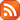Subscribe by RSS

# Operator Algebra Seminars

Fri., Nov. 2, 2018 1:30 p.m. - Fri., Nov. 2, 2018 2:30 p.m.

Location: Classroom Building (CL) 416

Speaker: Brian Ketelboeter

Title: C*-Convolutive Systems II

﻿Abstract:

A $C^*$-quantum convolutive system consists of a family of $C^*$-algebras $\{\mathcal{A}_{s,t}\}$,
given for all $s, t \in\mathbb{R}$, $s < t$, along with morphisms $\Delta_{r,s,t}: \mathcal{A}_{r,t}\to \mathcal{A}_{r,s}\otimes \mathcal{A}_{s,t}$ given for all
$r, s, t \in\mathbb{R}$, $r < s < t$, satisfying the co-associativity condition:
\begin{eqnarray*}\label{qcs}\left(\operatorname{id}_{\mathcal{A}_{r,s}}\otimes \Delta_{s,t,u}\right)\Delta_{r,s,u}=
\left( \Delta_{r,s,t}\otimes \operatorname{id}_{\mathcal{A}_{t,u}} \right)\Delta_{r,t,u}\end{eqnarray*}
whenever $r, s, t, u\in\mathbb{R}$, $r < s < t < u$.

We continue our discussion of convolutive systems; including the definitions and our first example.